# BTC, ETH, STEEM and HIVE analysis using Python (from 2020 to 2021)

in Programming & Dev2 years ago

Crypto Market study of BTC, ETH, STEEM and HIVE, from 2020 to 2021 (one full year). The main goal of this analysis, is to make a comparison of these four cryptocurrencies, using several python libraries and statistical analysis.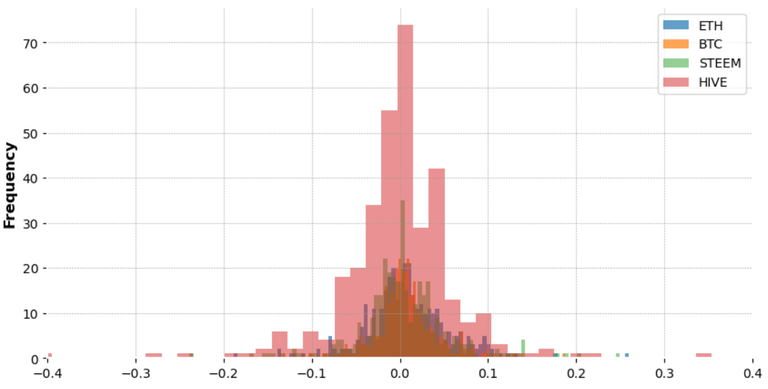##### Import Python Libraries
``````import pandas as pd
from datetime import datetime
import numpy as np
import seaborn as sns
from plotly.offline import iplot
import plotly.express as px
import matplotlib.pyplot as plt
cf.go_offline()
%config InlineBackend.print_figure_kwargs = {'bbox_inches':None}
%config InlineBackend.rc = {'font.size': 10, 'figure.figsize': (10.0, 7.0), 'figure.facecolor': (1, 1, 1, 0), 'figure.subplot.bottom': 0.125, 'figure.edgecolor': (1, 1, 1, 0), 'figure.dpi': 72}
%matplotlib inline
``````
##### Define start and end date
``````start = datetime(2020, 3, 30)
end = datetime(2021, 3, 24)
``````
##### Create Data Frames for each Cryptocurrency
``````BTC = web.DataReader('BTC-USD', 'yahoo', start, end)
ETH = web.DataReader('ETH-USD', 'yahoo', start, end)
STEEM = web.DataReader('STEEM-USD', 'yahoo', start, end)
HIVE = web.DataReader('HIVE-USD', 'yahoo', start, end)

cryptos = [BTC, ETH, STEEM, HIVE]

ticks = ['BTC', 'ETH', 'STEEM', 'HIVE']
``````
``````    High    Low Open    Close   Volume  Adj Close
Date
2020-03-29  0.214535    0.181311    0.193561    0.193029    37124.0 0.193029
2020-03-30  0.198990    0.164887    0.192400    0.178775    35451.0 0.178775
2020-03-31  0.203500    0.169290    0.178704    0.197060    35316.0 0.197060
2020-04-01  0.205896    0.170124    0.196492    0.182773    45439.0 0.182773
2020-04-02  0.191577    0.172460    0.182715    0.179288    60969.0 0.179288
``````
##### Concatenate Data Frames for better visualization
``````cryptos_df = pd.concat(cryptos, axis = 1, keys = ticks)
cryptos_df.columns.names = ['Cryptos', 'Info']
``````
##### Plots of "Open", "Close", "High" and "Low" for each Cryptocurrency
``````for i in ticks:
cryptos_df[i].drop(['Volume', 'Adj Close'], axis = 1).plot()
plt.title(i)
``````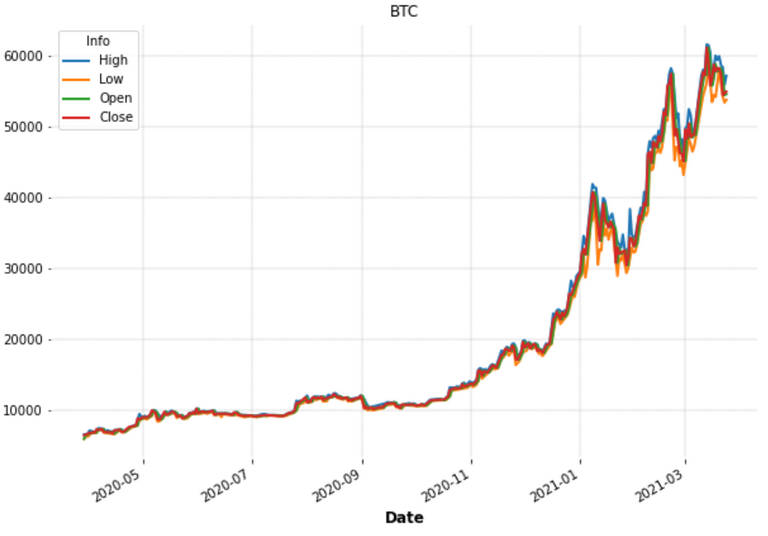##### Volume of each cryptocurrency
``````volumes = pd.DataFrame()

for i in ticks:
volumes[i] = cryptos_df[i]['Volume']

volumes[ticks[:2]].iplot()
volumes[ticks[2:]].iplot()
``````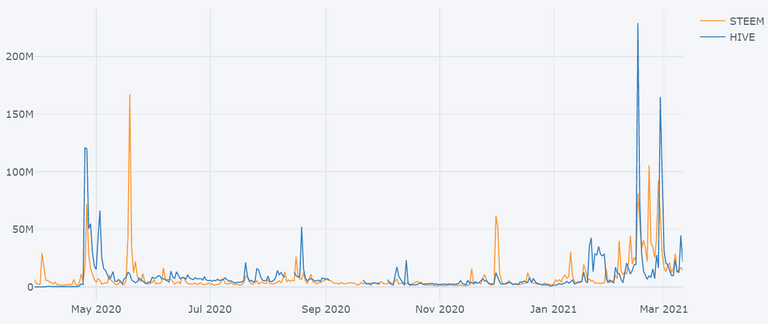##### Obtain dates for the highest volumes
``````max_vols = []
for i in ticks:
value = volumes[i].iloc[[volumes[i].argmax()]].to_frame()
max_vols.append(value)

high_volume = pd.concat(max_vols, axis = 1)

``````
DateVolume
BTC2021-02-263.509679e+11
ETH2021-01-116.073363e+10
STEEM2021-03-22475655991.0
HIVE2021-03-23846450368.0

We can see that both HIVE and STEEM had the highest volumes on this month (March 2021)

##### HIVE Candlestick Chart of 2021
``````import mplfinance as fplt
#from matplotlib.dates import DateFormatter, date2num, WeekdayLocator, DayLocator, MONDAY

fplt.plot(
HIVE.iloc[250:],
type='candle',
style='charles',
title='HIVE Candlestick (2021)',
ylabel='Price (\$)',
)
``````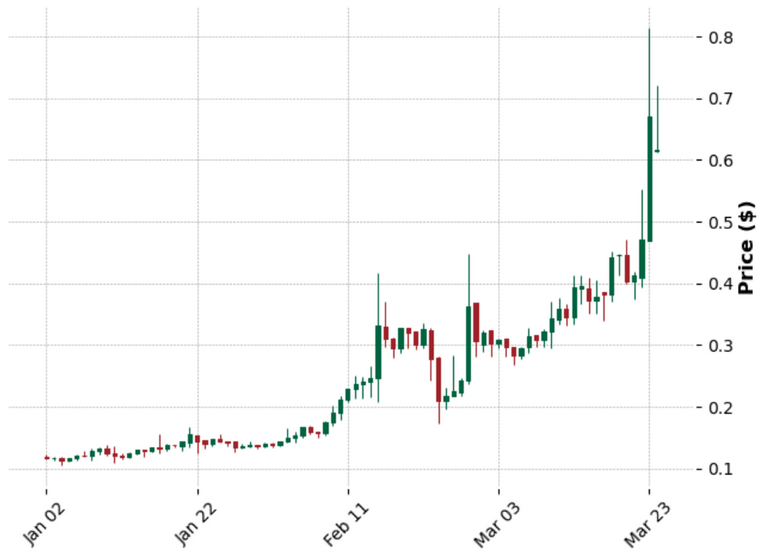##### Daily Percentage Change (Returns)

r = (P1/P2) - 1

``````returns = pd.DataFrame()

for i in ticks:
returns[i + ' Returns'] = cryptos_df[i]['Close'].pct_change()

``````
``````BTC Returns ETH Returns STEEM Returns   HIVE Returns
Date
2020-03-29  NaN NaN NaN NaN
2020-03-30  0.001369    0.005184    -0.026310   -0.073844
2020-03-31  0.026113    0.015278    0.021583    0.102279
2020-04-01  0.028281    0.047146    0.001803    -0.072501
2020-04-02  -0.008867   0.000438    0.140814    -0.019067
``````
###### Obtain maximum returns for each crypto
``````for i in range(len(ticks)):
print('Date: ' + str(returns[ticks[i] + ' Returns'].idxmax())[:10] + " Max Return " + ticks[i] + ': ' + str(returns.iloc[:, i].max()))

Date: 2021-02-08 Max Return BTC: 0.18746473766529936
Date: 2021-01-03 Max Return ETH: 0.2594753293956862
Date: 2021-03-22 Max Return STEEM: 0.32037727117672854
Date: 2020-04-23 Max Return HIVE: 1.3697716791032435
``````
###### Obtain worst returns for each crypto
``````returns.idxmin() # worst single day returns

BTC Returns     2021-01-21
ETH Returns     2021-01-21
STEEM Returns   2021-02-23
HIVE Returns    2020-04-26
``````

Even though the coronavirus outbreak had a great impact in the single day returns, the highest and the minimum values for BTC, ETH, and STEEM were already in 2021, probably due to the beginning of the bull market. HIVE is the exception, the best and worst single day returns occurred in April 2020, when HIVE spiked due to the STEEM fork

###### Moving average on HIVE around the maximum returns
``````HIVE['Returns'].argmax()

24

HIVE['MA'] = HIVE.iloc[:60]['Returns'].rolling(3).mean() # smoothing with Moving Average
``````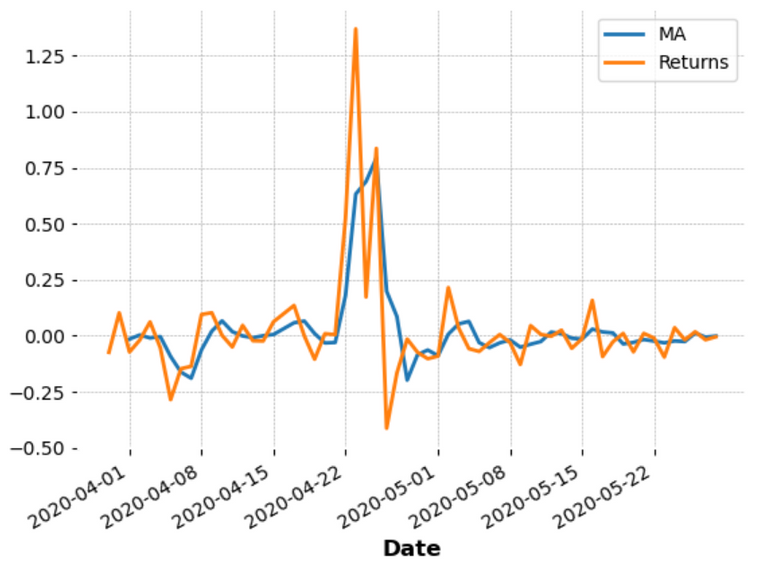##### Volatility
``````fig = plt.figure(figsize=(10, 5))

returns['ETH Returns'].plot.hist(bins = 100, label = 'ETH', alpha = 0.7)
returns['BTC Returns'].plot.hist(bins = 100, label = 'BTC', alpha = 0.7)
returns['STEEM Returns'].plot.hist(bins = 100, label = 'STEEM', alpha = 0.5)
returns['HIVE Returns'].plot.hist(bins = 100, label = 'HIVE', alpha = 0.5)
plt.xlim([-0.4, 0.4])
plt.legend(fontsize = 10)
``````From the histograms above, we can see that all the cryptocurrencies are volatile because they show a big deviation. Nevertheless, HIVE is the one with higher volatility in the defined range. This volatility, might be related to the STEEM fork, some days after HIVE's launch, the price peaked to ATH very rapidly.

``````fig = plt.figure(figsize=(10, 5))

returns['BTC Returns'].plot(kind = 'kde', label = 'BTC')
returns['ETH Returns'].plot(kind = 'kde', label = 'ETH')
returns['STEEM Returns'].plot(kind = 'kde', label = 'STEEM')
returns['HIVE Returns'].plot(kind = 'kde', label = 'HIVE')
plt.xlim([-0.2, 0.2])
plt.legend(fontsize = 10)
``````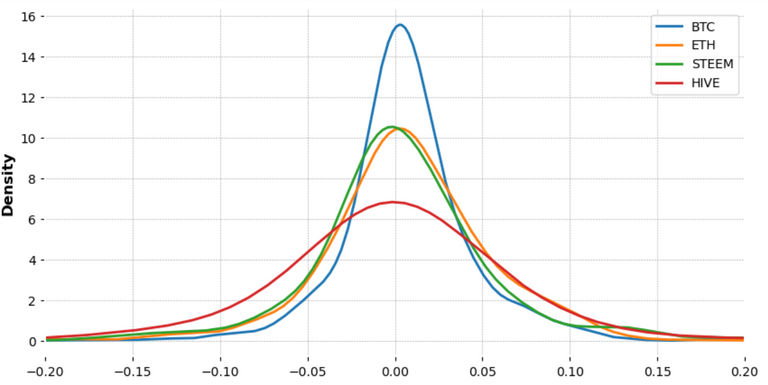The kernel plots above confirm that HIVE is the one with higher volatility. Easily confirmed by the large distribution. BTC is the less volatile from the following cryptocurrencies, in the defined range.

###### Standard deviation of the Returns
``````returns.std()

BTC Returns      0.035037
ETH Returns      0.047000
STEEM Returns    0.056024
HIVE Returns     0.116130
dtype: float64
``````

For this year only...

``````returns.loc['2021-01-01':].std()

BTC Returns      0.049388
ETH Returns      0.063443
STEEM Returns    0.080352
HIVE Returns     0.102327
dtype: float64
``````

This year HIVE keeps on showing higher risk compared to the other cryptos. However, it is the youngest crypto of the four, so more risk/reward associated, but at the same time, more space to grow.

Sort:

I should look into this :) thank you for sharing it . I had totally forgotten about the standard deviation function so yeah this will help .

Posted Using LeoFinance Beta

Congratulations @macrodrigues! You have completed the following achievement on the Hive blockchain and have been rewarded with new badge(s) :You received more than 10 upvotes.Your next target is to reach 50 upvotes.

If you no longer want to receive notifications, reply to this comment with the word `STOP`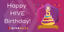Happy Birthday to the Hive Community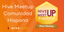A successful meetup and its commemorative badge